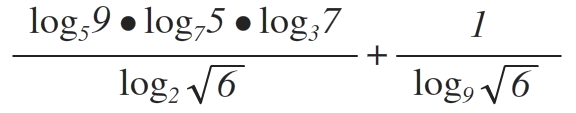# How would you Handle The Ever-changing Logarithm? Part 1

Algebra Level 3Imagine you are John Napier who arrived into this present future from the past, who was also credited for attributing to the success of deriving the concept of Logarithms and found answers to bizarre questions like $N ={ 10 }^{ 7 }\left( 1-{ 10 }^{ 7 } \right) ^{ L }$ and introduced the letter L as 'Logarithm'.

One Day, a mathematician approached him and was asking everyone on the street to evaluate this.....(above the passage).

Details: John was only given a pencil and an A4 piece of paper by the mathematician to attempt on the question and got the value of it quickly. The mathematician soon gave him a job and both continued to make great contributions in logarithms. (Do not use calculator to solve it)

×